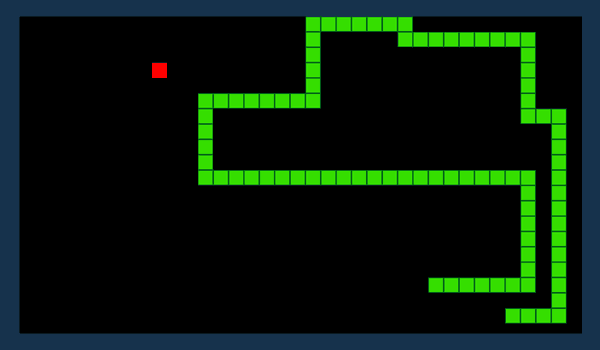# Snake's NeckmainstringLU CSE Carnival 2019 Inte...
Limits 1s, 512 MB

Remember Violet and Dash from the movie "The Incredibles"? The two siblings recently installed the classic Snake Game in their phones and started competing each other. Soon they got bored and found a new competition in this game. "Let's find the largest similar contiguous subsequence of our snakes!". But they got stuck in there. Can you help them?The board of this game is a simple grid of $N \times M$ size. A cell of this board is represented by it's row number ($X$) and column number ($Y$) in $[X, Y]$ format. Two cells in this board are adjacent if they share a common side. The Snake is represented by some adjacent cells of this board. Each board contains exactly one snake. The snake's consecutive cells are guaranteed to be adjacent in the board. All cells of a snake are unique.

You are given two such snakes from two different games. You have find the largest similar contiguous subsequence (consecutive cells of the subsequence will have to adjacent in snake's body) of the given snakes. Two subsequence will be similar if all of their cell coordinates match in exact order.

## Input

The first line of the input contains one integer $L1$ (length of the first snake). Then $L1$ pairs of integers $(X, Y)$ follow. Each pair denoting one cell of the first snake.

The next line of the input contains one integer $L2$ (length of the second snake). Then $L2$ pairs of integers $(X, Y)$ follow. Each pair denoting one cell of the second snake.

Constraints:

• $2 \le L1, L2 \le 10^5$

• $0 \le X, Y \le10^4$

## Output

Print one integer. The length of the largest similar contiguous subsequence of two snakes.

## Samples

InputOutput
5
1 1
1 2
1 3
1 4
1 5
9
3 2
2 2
1 2
1 3
0 3
0 4
1 4
2 4
3 4

2
InputOutput
5
2 2
3 2
4 2
5 2
6 2
7
2 5
3 5
4 5
5 5
6 5
7 5
8 5

0
InputOutput
5
1 1
1 2
1 3
1 4
1 5
9
3 4
2 4
1 4
0 4
0 3
1 3
1 2
2 2
3 2

2

Yes, you can match in reverse order too! Because its a snake.DP

### Submit